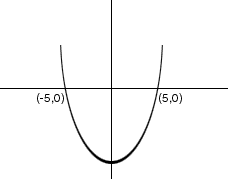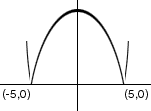Sender: corrie Subject: calculus question hi! i have a question i was hoping you could help me with! I need to find if the mean value theorem exists. and if so, find all values c guaranteed by the theorem. f(x) = |x2-25| on the interval [-10,0] thanks for your time and help! corrie secondary student Hi Corrie, For the Mean Value Theorem to apply you need f(x) differentiable in the interval (-10, 0). My first thought is to sketch the graph of the function f(x) = |x2-25| The function g(x) = x2-25 is a parabola that opens upward and crosses the X-axis at the points where x2-25 = 0, that is where x = 5 and x = -5. The graph isy = x2-25 When you take the absolute value of the y-values to get the function f(x), the negative y-values become positive. Thus the part of the graph below the X-axis gets "turned over" and the graph becomesy = |x2-25| At the point (-5, 0), which is inside the interval (-10, 0) the curve clearly has no tangent line. Thus y = f(x) is not differentiable at x = -5 and thus the Mean Value Theorem does not apply. Cheers, Harley Go to Math Central